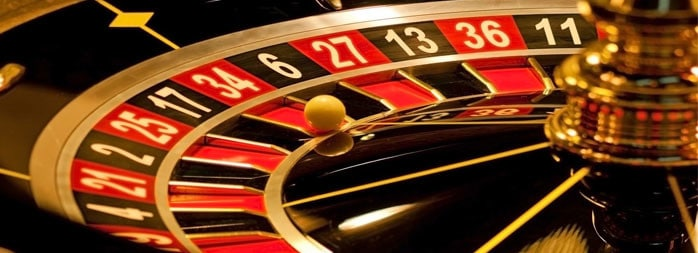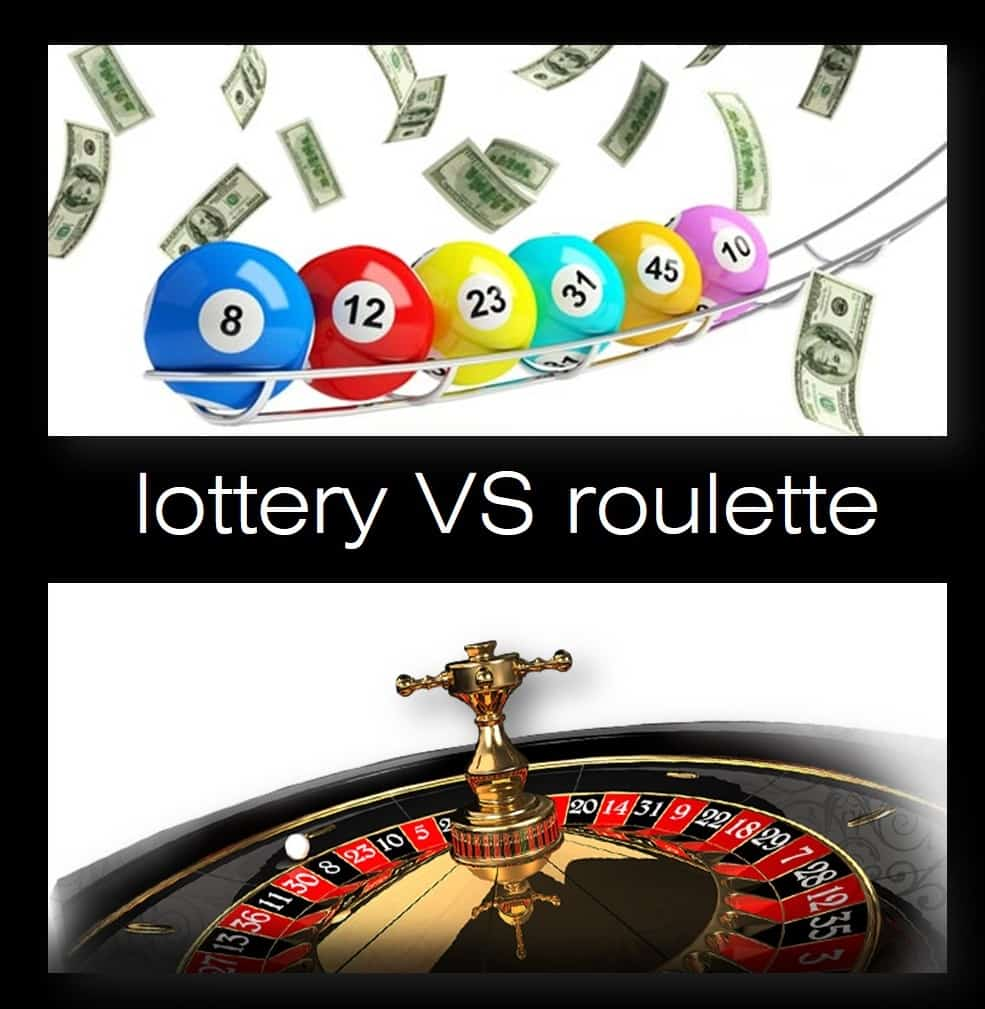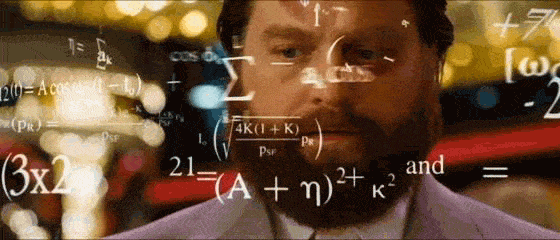# How Math Works in GamblingGambling was the main reason for the emergence and development of the probability theory. Like any other mathematical theory, it sets its own laws. It seems strange indeed that any case may be regulated. Consequently, knowing the rules, can we win a random game? Let’s see.

The first thing to clarify is the notion of the random game itself; it is the one where a player cannot have any influence on the game’s outcome. Chess, bridge or sports games aren’t random as opposed to tossing coins and roulette.

### Mathematical expectation

In some games, like an ordinary bingo lottery, the player doesn’t actually take part (except for the ticket purchase). Other games, say, in the casino — roulette and blackjack — allow more active participation of the player, who can control the rates and the game type. In brief, the smaller player involvement leads to the greater prize.In any case, we have a clear understanding that the casino always wins because, from a mathematical point of view, this game is not fair. The concept of a fair game is closely linked to the mathematical expectation. In a game, where a probability of events is known, the mathematical expectation represents the average prize per game, denoted by the letter $E$. The game is considered fair when the expectation equals zero. Let’s look at the example of how to find the mathematical expectation.

Suppose that someone suggests you the following: throw the dice; if the point is $1$ , you pay $$30$, and if something else, then you win$ $4$. First thing you need is to calculate the probability of each event. Probability $P (1)$ (that $1$ will come up) equals $\frac {1}{6}$ (one favorable case of six possible), and the probability of any other number outcomes is $\frac {5}{6}$. The mathematical expectation is calculated as the sum of all probabilities, multiplied by the corresponding incomes or losses (income is taken with the “plus” sign, loss is taken with the “minus”  sign). In this case, the mathematical expectation is equal to

$E=4\cdot \frac{5}{6}-30\cdot \frac{1}{6}= – \frac{10}{6}= – 1,66$ dollars.

This is the sum of the average income, which our opponent will receive if we agree to play. This game will be fair if we receive $6$ dollars in the case anything except $1$ comes up:

$E=6\cdot \frac{5}{6}-30\cdot \frac{1}{6}=\frac{30}{6}-\frac{30}{6}=5-5=0$

Sometimes intuition can help to determine whether the game is favorable, unfavorable or unfair, but there are many situations in wich this intuition isn’t a useful tool and it appears better to use a pencil and a paper. There are many examples showing how misleading it can be. For instance, in the meeting, which involves $23$ people, the probability of finding two people with the same date of birth is slightly higher than the probability of the heads outcome in coin tossing.

Here’s another example. Suppose two players, $A$ and $B$, play the following game: player $A$ randomly picks a card from the deck of $36$  cards. If player B has a jack, a queen and a king, he has to pay $300; if he has an ace, then he pays$2 to player A, who also has to pay \$25 to player B. Who wins?First, we should find the probability of each outcome. The deck has $36$ cards, which include only $12$ jacks, kings and queens, so the probability of that one of these cards comes up is:

$P_{f}=\frac {12}{36}=\frac {1}{3}$

Since there are only $4$ aces, the probability of their outcome is

$P_a=\frac{4}{36}=\frac{1}{9}$

Let’s exclude the jacks, queens, kings and aces from the deck so that there would be $20$ cards left, so the probability of pulling out a card, which is different from the above:

$P=\frac{20}{36}=\frac{5}{9}$

Now, we can apply the formula for the calculation of the mathematical expectation of this game

$E=300\cdot P_{f}-2\cdot P_{a}-25\cdot P$,

$E=300\cdot \frac{1}{3}-2\cdot \frac{1}{9}-25\cdot \frac{5}{9}$,

$E=\frac{773}{9} \approx 85.89$.

This is the average player’s profit  $A$.

Overall, the game is obviously not fair, so if you want to make a money in the casino, it’s better to open your own casino! By the way, here is one of the luckiest and craziest casino winners, who put his whole life on one roulette spin.

4 Shares
Filed under Math.